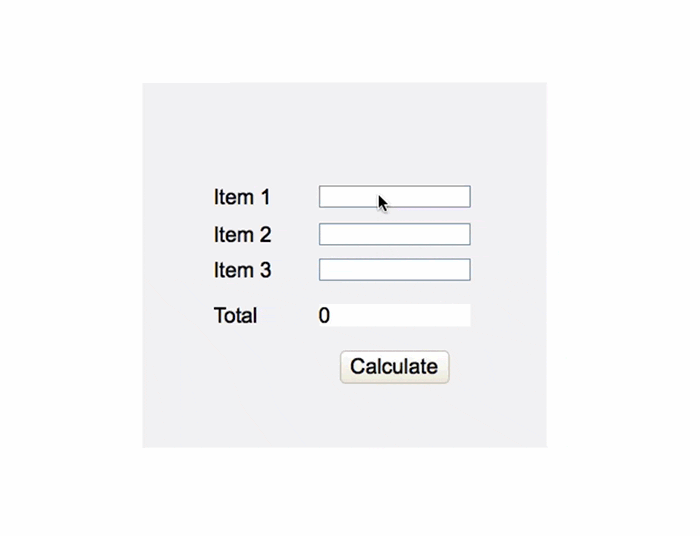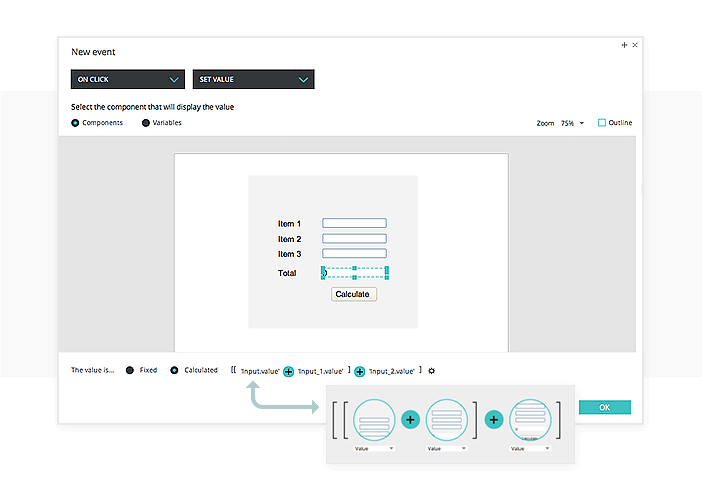# Value calculations for your prototypes

Add mathematical expressions to your prototypes. In our example, the total of three numerical values you enter are calculated when you press the “Calculate” button.3. In the builder, drag the first three Input Text Fields and the ‘+’ function into the expression, as shown in the image below: